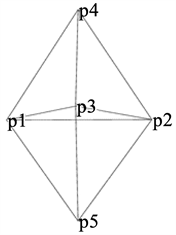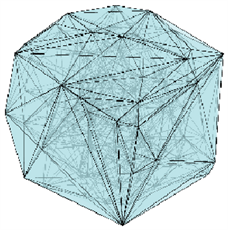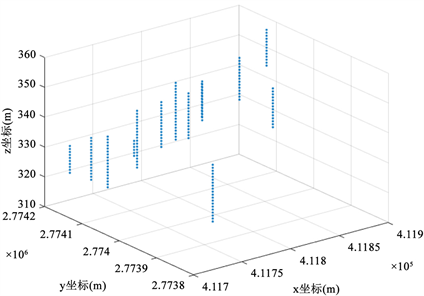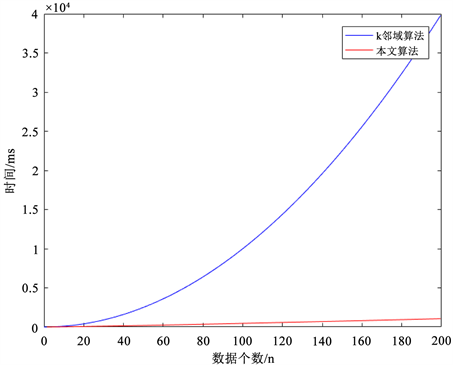# 基于Delaunay三角剖分的空间离群点检测算法研究Spatial Outlier Detection Based on TEN Mod-el and Weighted Attribute

• 全文下载: PDF(844KB)    PP.1-8   DOI: 10.12677/CSA.2019.91001
• 下载量: 349  浏览量: 502

A Delaunay triangulation based spatial outlier detection algorithm is proposed to solve the problems of the traditional spatial outlier detection algorithm, such as the high complexity of the algorithm and the large human influence on the construction of spatial neighbors. In this algorithm, Delaunay triangulation is carried out on the spatial data points to establish the spatial neighbor-hood. Based on the generated spatial neighborhood, outlier factors in the spatial neighborhood are defined according to SLOF algorithm, combined with the specific drilling data of a mine in a certain area. Experimental results show that this algorithm can effectively detect spatial outliers with low complexity and low human influence.

1. 引言

Shekhar等人从空间数据的本质出发，首次提出了二分法(SLZ)  ，将空间数据分为空间属性和非空间属性，根据空间属性确定空间对象的空间邻域，通过计算非空间属性确定空间对象邻域内的差异，计算离群因子。但是SLZ算法使用的是全局的阈值，求出的是全局的空间离群点。

2. 基于Delaunay三角剖分的空间离群检测算法Figure 1. Spatial outlier detection algorithm based on Delaunay triangulation

2.1. 相关定义

$dist\left({s}_{i},{s}_{j},w\right)=\sqrt{{{\sum }_{k=1}^{d}{w}_{k}\left(f\left({s}_{{i}_{k}}\right)-f\left({s}_{{j}_{k}}\right)\right)}^{2}}$ (公式2.1)

$Ndist\left(s,w\right)=\frac{{\sum }_{p\in NB\left(s\right)}dist\left(p,s,w\right)}{|NB\left(s\right)|}$ (公式2.2)

$\text{SLOF}\left(s\right)=\frac{Ndist\left(s,w\right)}{\frac{{\sum }_{p\in NB\left(o\right)}Ndist\left(s,w\right)}{|NB\left(o\right)|}}$ (公式2.3)

2.2. 数据预处理

2.3. 通过Delaunay三角剖分构建空间邻域

2.4. 建立空间权重矩阵Table 1. Generating spatial neighborhood table based on Delaunay triangulation

2.5. 空间邻域离群因子的度量

$\text{SLOF}\left(s\right)=\frac{Ndist\left(s,w\right)+\delta }{\frac{{\sum }_{p\in NB\left(s\right)}Ndist\left(s,w\right)}{|NB\left(s\right)|}+\delta }$ (公式2.4)

$\delta$ 一般取值为1 * 10−6左右，当SLOF的值大于1时，离群程度开始变高，当小于1时，表示离群程度正常。最终选取SLOF值前n个大的点作为空间离群点。(a) 空间对象示意(b) 基于Delaunay三角剖分生成的空间权重矩阵

Figure 2. Schematic diagram of spatial weight matrix

2.6. 算法的描述与复杂度分析

Step 1：对空间数据集进行预处理，甄选出全局离群点，需要求取数据集合的均值方差等，时间复杂度为 $O\left(N\right)$

Step 2：建立空间邻域，通过Delaunay三角剖分对空间数据集进行处理，建立空间邻域，时间复杂度为 $O\left(N\mathrm{log}N\right)$

Step 3：计算空间权重矩阵，利用Delaunay三角剖分生成的空间邻域，将全局离群点灯值用邻域均值代替，最后建立空间权重矩阵，时间复杂度为 $O\left(N\mathrm{log}N\right)$

Step 4：计算空间邻域内的空间离群因子，结合SLOF算法，根据生成的空间邻域矩阵生成空间离群因子，时间复杂度为 $O\left(N\mathrm{log}N\right)$

3. 实验和分析

3.1. 算法实验(a) 钻孔采样点散点图(b) 空间邻域示意图

Figure 3. Scatter diagram and spatial neighborhood diagram of borehole sampling pointsFigure 4. Comparison of time complexity between the two algorithms

3.2. 实验结果分析

1) 从检测精度对两种方法进行分析：结合现场采集数据具体情况，可以发现基于Delaunay三角剖分方法检测出的离群点，其检测精度达到80%，而k邻域的方法，其检测精度仅在k值为5时，最高达到80%，因此可以得出本文所提算法的检测精度高于k邻域方法。Table 2. Outliers detected from Delaunay triangulationTable 3. SLOF algorithm detects different k values

2) 从人为因素进行分析：k邻域的算法，在k值选取方面存在人为因素，最优k值难以选择。而本文算法，完全基于Delaunay三角剖分建立空间邻域，无需对k值进行设定和检测。

3) 从算法时间复杂度进行分析：如图4所示，当数据量增长较快时，本文算法时间复杂度增长较为缓慢，而k邻域算法时间复杂度增长呈指数增长，因此可得本文算法在时间复杂度方面优于k邻域算法。

4. 结束语

  Chen, D., Lu, C.T., Kou, Y., et al. (2008) On Detecting Spatial Outliers. Geoinformatica, 12, 455-475. https://doi.org/10.1007/s10707-007-0038-8  Wackernagel, H. (2003) Variogram Cloud. Multivariate Geostatistics. https://doi.org/10.1007/978-3-662-05294-5_6  Das, B.R.S.I. and Plots, Q.Q. (2008) Random Sets and Data from a Heavy Tailed Distribution. Communications in Statistics Stochastic Models, 24, 103-132. https://doi.org/10.1080/15326340701828308  Anselin, L. (2003) Spatial Externalities, Spatial Multipliers, and Spatial Econometrics. International Regional Science Review, 26, 153-166. https://doi.org/10.1177/0160017602250972  Shekhar, S., Lu, C. and Zhang, P. (2003) A Unified Approach to Detecting Spatial Outliers. Geoinformatica, 7, 139-166. https://doi.org/10.1023/A:1023455925009  Shekhar, S., Lu, C. and Zhang, P. (2003) A Unified Approach to Detecting Spa-tial Outliers. Geoinformatica, 7, 139-166. https://doi.org/10.1023/A:1023455925009  文俊浩, 吴中福, 吴红艳. 空间孤立点检测[J]. 计算机科学, 2006(5): 186-187 + 210.  Chawla, S. and Sun, P. (2006) SLOM: A New Measure for Local Spatial Outliers. Springer-Verlag, New York.  薛安荣, 鞠时光, 何伟光, 等. 局部离群点挖掘算法研究[J]. 计算机学报, 2007, 30(8): 1455-1463.  张忠平, 徐晓云, 王培. 基于KNN图的空间离群点挖掘算法[J]. 计算机工程, 2011, 37(4): 37-39.